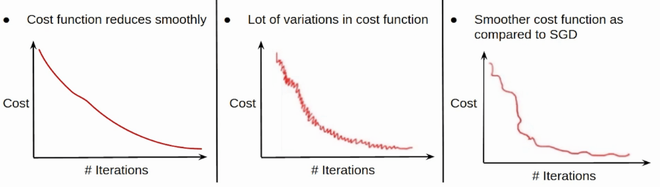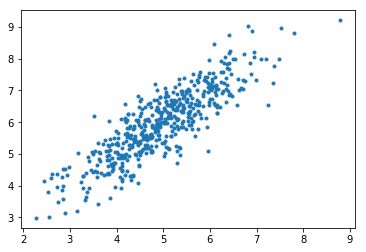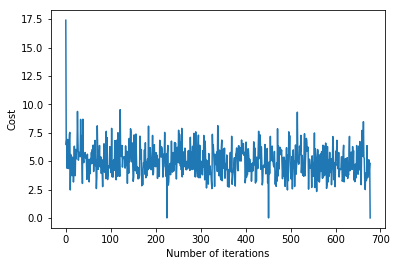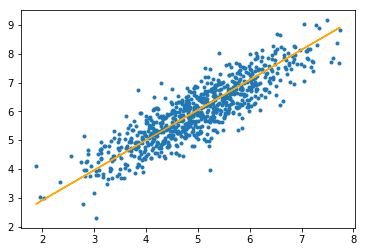GeeksforGeeks App
Open AppBrowser
Continue

# ML | Mini-Batch Gradient Descent with Python

In machine learning, gradient descent is an optimization technique used for computing the model parameters (coefficients and bias) for algorithms like linear regression, logistic regression, neural networks, etc. In this technique, we repeatedly iterate through the training set and update the model parameters in accordance with the gradient of the error with respect to the training set. Depending on the number of training examples considered in updating the model parameters, we have 3-types of gradient descents:

1. Batch Gradient Descent: Parameters are updated after computing the gradient of the error with respect to the entire training set
2. Stochastic Gradient Descent: Parameters are updated after computing the gradient of the error with respect to a single training example
3. Mini-Batch Gradient Descent: Parameters are updated after computing the gradient of  the error with respect to a subset of the training set

Thus, mini-batch gradient descent makes a compromise between the speedy convergence and the noise associated with gradient update which makes it a more flexible and robust algorithm.Convergence in BGD, SGD & MBGD

Let theta = model parameters and max_iters = number of epochs. for itr = 1, 2, 3, …, max_iters:       for mini_batch (X_mini, y_mini):

• Forward Pass on the batch X_mini:
• Make predictions on the mini-batch
• Compute error in predictions (J(theta)) with the current values of the parameters
• Backward Pass:
• Compute gradient(theta) = partial derivative of J(theta) w.r.t. theta
• Update parameters:
• theta = theta – learning_rate*gradient(theta)

Below is the Python Implementation:

Step #1: First step is to import dependencies, generate data for linear regression, and visualize the generated data. We have generated 8000 data examples, each having 2 attributes/features. These data examples are further divided into training sets (X_train, y_train) and testing set (X_test, y_test) having 7200 and 800 examples respectively.

## Python3

 `# importing dependencies``import` `numpy as np``import` `matplotlib.pyplot as plt` `# creating data``mean ``=` `np.array([``5.0``, ``6.0``])``cov ``=` `np.array([[``1.0``, ``0.95``], [``0.95``, ``1.2``]])``data ``=` `np.random.multivariate_normal(mean, cov, ``8000``)` `# visualising data``plt.scatter(data[:``500``, ``0``], data[:``500``, ``1``], marker``=``'.'``)``plt.show()` `# train-test-split``data ``=` `np.hstack((np.ones((data.shape[``0``], ``1``)), data))` `split_factor ``=` `0.90``split ``=` `int``(split_factor ``*` `data.shape[``0``])` `X_train ``=` `data[:split, :``-``1``]``y_train ``=` `data[:split, ``-``1``].reshape((``-``1``, ``1``))``X_test ``=` `data[split:, :``-``1``]``y_test ``=` `data[split:, ``-``1``].reshape((``-``1``, ``1``))` `print``(& quot``       ``Number of examples ``in` `training ``set``=` `%` `d & quot``       ``%` `(X_train.shape[``0``]))``print``(& quot``       ``Number of examples ``in` `testing ``set``=` `%` `d & quot``       ``%` `(X_test.shape[``0``]))`

Output:Number of examples in training set = 7200 Number of examples in testing set = 800

Step #2: Next, we write the code for implementing linear regression using mini-batch gradient descent. gradientDescent() is the main driver function and other functions are helper functions used for making predictions – hypothesis(), computing gradients – gradient(), computing error – cost() and creating mini-batches – create_mini_batches(). The driver function initializes the parameters, computes the best set of parameters for the model, and returns these parameters along with a list containing a history of errors as the parameters get updated.

Example

## Python3

 `# linear regression using "mini-batch" gradient descent``# function to compute hypothesis / predictions`  `def` `hypothesis(X, theta):``    ``return` `np.dot(X, theta)` `# function to compute gradient of error function w.r.t. theta`  `def` `gradient(X, y, theta):``    ``h ``=` `hypothesis(X, theta)``    ``grad ``=` `np.dot(X.transpose(), (h ``-` `y))``    ``return` `grad` `# function to compute the error for current values of theta`  `def` `cost(X, y, theta):``    ``h ``=` `hypothesis(X, theta)``    ``J ``=` `np.dot((h ``-` `y).transpose(), (h ``-` `y))``    ``J ``/``=` `2``    ``return` `J[``0``]` `# function to create a list containing mini-batches`  `def` `create_mini_batches(X, y, batch_size):``    ``mini_batches ``=` `[]``    ``data ``=` `np.hstack((X, y))``    ``np.random.shuffle(data)``    ``n_minibatches ``=` `data.shape[``0``] ``/``/` `batch_size``    ``i ``=` `0` `    ``for` `i ``in` `range``(n_minibatches ``+` `1``):``        ``mini_batch ``=` `data[i ``*` `batch_size:(i ``+` `1``)``*``batch_size, :]``        ``X_mini ``=` `mini_batch[:, :``-``1``]``        ``Y_mini ``=` `mini_batch[:, ``-``1``].reshape((``-``1``, ``1``))``        ``mini_batches.append((X_mini, Y_mini))``    ``if` `data.shape[``0``] ``%` `batch_size !``=` `0``:``        ``mini_batch ``=` `data[i ``*` `batch_size:data.shape[``0``]]``        ``X_mini ``=` `mini_batch[:, :``-``1``]``        ``Y_mini ``=` `mini_batch[:, ``-``1``].reshape((``-``1``, ``1``))``        ``mini_batches.append((X_mini, Y_mini))``    ``return` `mini_batches` `# function to perform mini-batch gradient descent`  `def` `gradientDescent(X, y, learning_rate``=``0.001``, batch_size``=``32``):``    ``theta ``=` `np.zeros((X.shape[``1``], ``1``))``    ``error_list ``=` `[]``    ``max_iters ``=` `3``    ``for` `itr ``in` `range``(max_iters):``        ``mini_batches ``=` `create_mini_batches(X, y, batch_size)``        ``for` `mini_batch ``in` `mini_batches:``            ``X_mini, y_mini ``=` `mini_batch``            ``theta ``=` `theta ``-` `learning_rate ``*` `gradient(X_mini, y_mini, theta)``            ``error_list.append(cost(X_mini, y_mini, theta))` `    ``return` `theta, error_list`

Calling the gradientDescent() function to compute the model parameters (theta) and visualize the change in the error function.

## Python3

 `theta, error_list ``=` `gradientDescent(X_train, y_train)``print``("Bias ``=` `", theta[``0``])``print``("Coefficients ``=` `", theta[``1``:])` `# visualising gradient descent``plt.plot(error_list)``plt.xlabel("Number of iterations")``plt.ylabel("Cost")``plt.show()`

Output: Bias = [0.81830471] Coefficients = [[1.04586595]]Step #3: Finally, we make predictions on the testing set and compute the mean absolute error in predictions.

## Python3

 `# predicting output for X_test``y_pred ``=` `hypothesis(X_test, theta)``plt.scatter(X_test[:, ``1``], y_test[:, ], marker``=``'.'``)``plt.plot(X_test[:, ``1``], y_pred, color``=``'orange'``)``plt.show()` `# calculating error in predictions``error ``=` `np.``sum``(np.``abs``(y_test ``-` `y_pred) ``/` `y_test.shape[``0``])``print``(& quot``       ``Mean absolute error ``=` `& quot``       ``, error)`

Output:Mean absolute error = 0.4366644295854125

The orange line represents the final hypothesis function: theta + theta*X_test[:, 1] + theta*X_test[:, 2] = 0

My Personal Notes arrow_drop_up